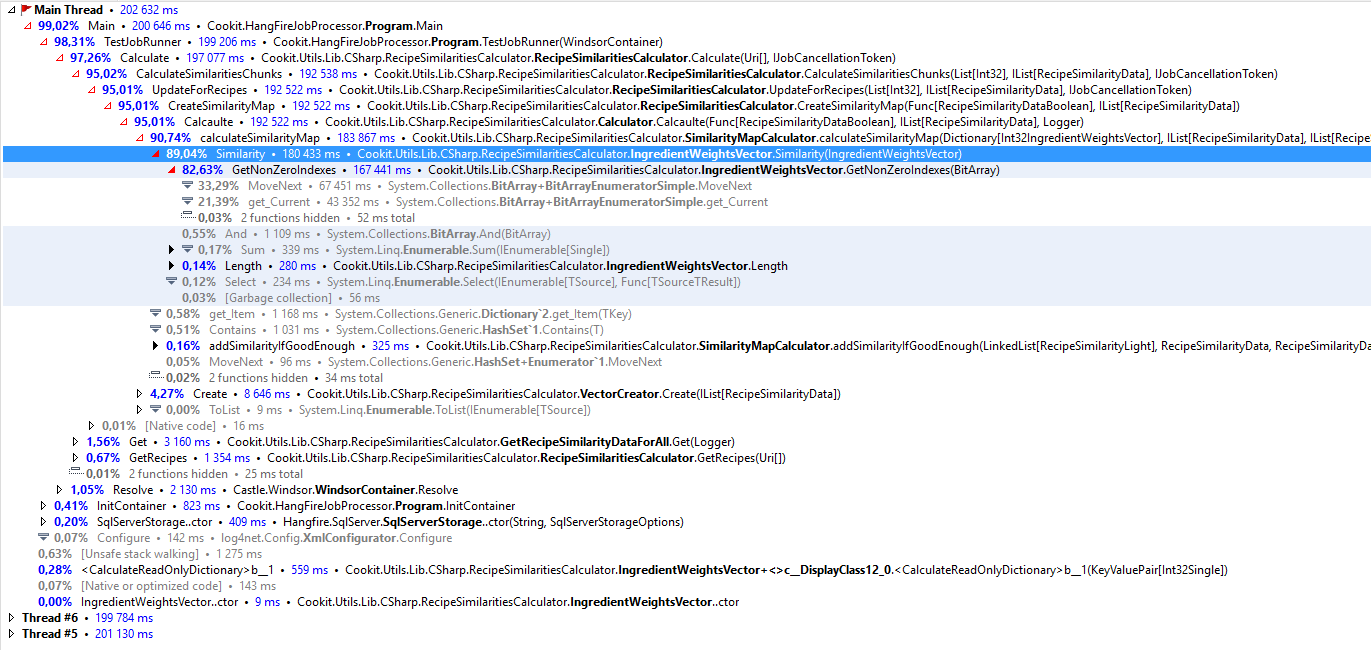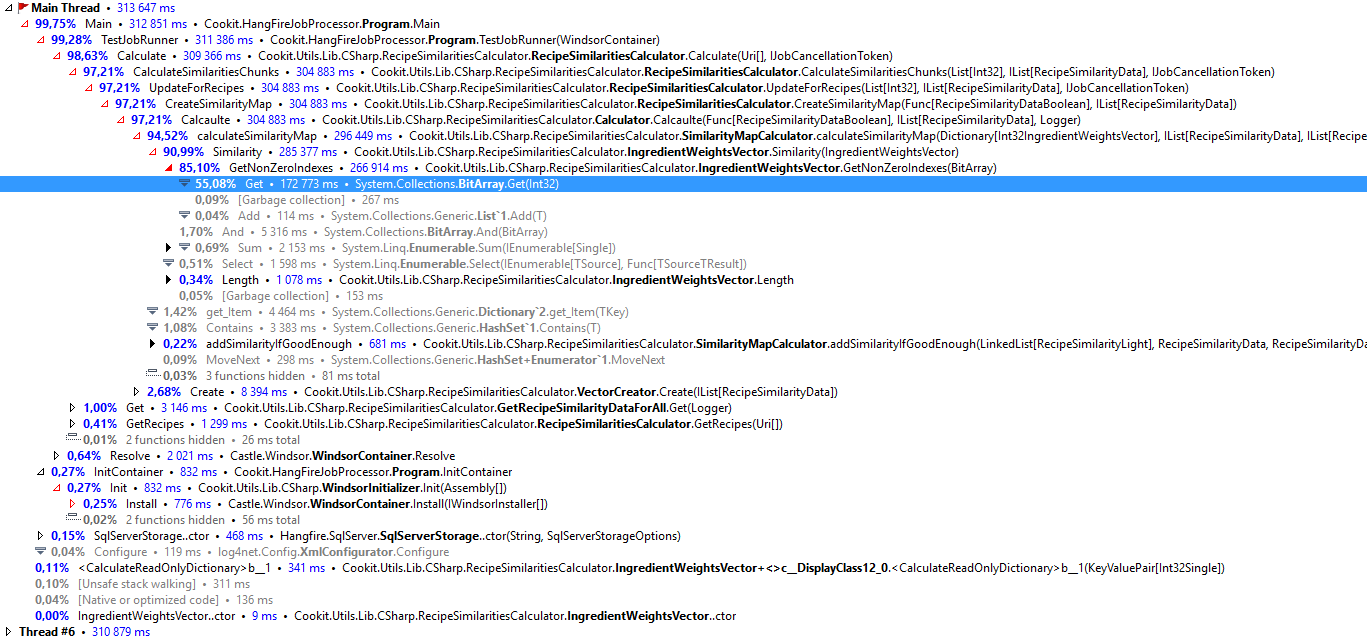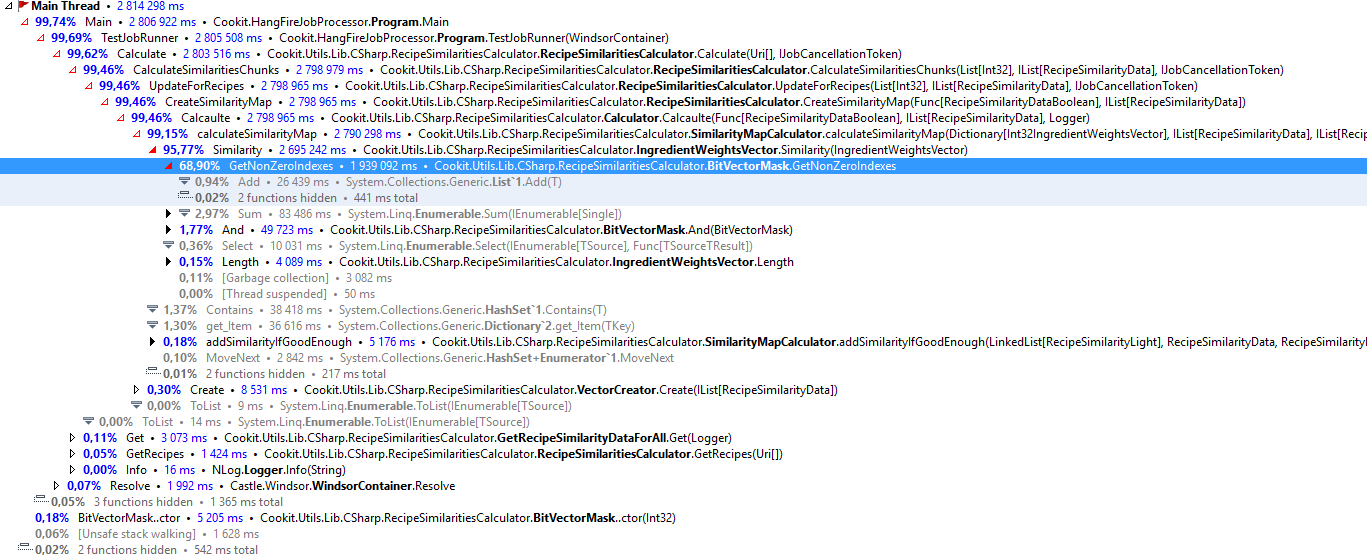# Using bit masks for high-performance calculations

## December 13, 2016

Last time I’ve shown how I’ve gone from 34 hours to 11. This time we go faster. To go faster I have to do less.

The current implementation of `Similarity` iterates over one vector and checks if that ingredient exists in the second one. Since those vectors are sparse the chance of a miss is big. This means that I am losing computational power on iterating and calling `TryGetValue`.

How to iterate only over the mutually owned ones and do it fast?

The fastest and least memory consuming way is to use an `int` or a `long` and set their bits individually. There is one problem with this. I need a mask for 1800 values, so my number would have to be 1800 bit… But before I go further why not use an array of bools? The memory overhead would be minimal (~1MB), but the cost of iterating would be significant. Don’t trust me? Keep on reading :)

### .NET’s BitArray

Luckily .NET has `BitArray` class and it implements the `AND` operation, so let’s give it a try:

``````public float Similarity(IngredientWeightsVector otherVector)
{
var nonZeros = GetNonZeroIndexes(andMask); // get the indexes of the ingredients
float num = nonZeros.Sum(t => otherVector.IngredientWeights[t]*IngredientWeights[t]);
var denom = Length()*otherVector.Length();
return num/denom;
}

private List<int> GetNonZeroIndexes(BitArray ba)
{
List<int> ret = new List<int>(20);
int index = 0;
foreach (bool b in ba)
{
if(b)
index++;
}
return ret;
}
``````

Lets walk through what is happening here.
I calculate the `AND` between the masks of both vectors. This gives me the mask of ingredients present in both recipes. Then, since I need the weights for those ingredients, I iterate over the mask and find indexes (`GetNonZeroIndexes` takes care of it). With them, I can get the weights from both recipes vectors. Having those weights I can finally calculate the dot product.

The code is ready, so let’s fire it up!

Sadly I won’t give you the numbers because I’ve decided to terminate the calculation when it passed the point when it became clear that it will be way slower than the first implementation. So let’s fire up the profiler and see what is happening:As I saw earlier when optimizing this problem - iteration is not something free :(
Maybe it’s `foreach` fault and a simple `for` will be OK? Let’s check:`Get` is the function called on the indexer. So is this a dead end? Before taking this decision lets look at the code of `Get` in the `BitArray`:

``````public bool Get(int index)
{
if (index < 0 || index >= Length)
{
throw new ArgumentOutOfRangeException(nameof(index), index, SR.ArgumentOutOfRange_Index);
}
Contract.EndContractBlock();

return (m_array[index / 32] & (1 << (index % 32))) != 0;
}
``````

What can I improve there?

• remove the ifs at the start, because I like my performance unchecked ;)
• `BitArray` is using array of `int` as storage and I think using a `long` be a bit faster

### Custom BitArray implementation

Lets try with a custom implementation:

``````using System;
using System.Collections;
using System.Collections.Generic;
using System.Runtime.CompilerServices;

namespace Cookit.Utils.Lib.CSharp.RecipeSimilaritiesCalculator
{
public class MyBitArray
{
public int Length;

public MyBitArray(int size)
{
Length = size;
var numberOfLongs = (int) Math.Ceiling(((float) size)/64);
_array = new long[numberOfLongs];
}

[MethodImpl(MethodImplOptions.AggressiveInlining)]
private void CalcIndex(int index, out int longIndex, out long masc)
{
longIndex = GetLongIndex(index);
var rest = index - longIndex*64;
}

[MethodImpl(MethodImplOptions.AggressiveInlining)]
private int GetLongIndex(int index)
{
return index/64;
}

[MethodImpl(MethodImplOptions.AggressiveInlining)]
internal static long GetMask(int bitnum, bool val)
{
if (val)
return (1L << bitnum);
return ~(1L << bitnum);
}

public void Set(int index, bool value)
{
var longIndex = 0;
long longMasc = 0;
CalcIndex(index, out longIndex, out longMasc);
var currentValue = _array[longIndex];
currentValue = currentValue | longMasc;
_array[longIndex] = currentValue;
}

{
for (var i = 0; i < _array.Length; i++)
{
ret._array[i] = _array[i] & other._array[i];
}
return ret;
}

public IList<int> GetNonZeroIndexes()
{
List<int> ret= new List<int>();
for (var i = 0; i < _array.Length; i++)
{
for (int j = 0; j < 64; j++)
{
if (IsBitSet(_array[i], j))
}
}
return ret;
}

[MethodImpl(MethodImplOptions.AggressiveInlining)]
static bool IsBitSet(long b, int pos)
{
return (b & (1L << pos)) != 0;
}
}
}
``````

Test run for the sample took 2509 sec, so the full run will take:

``````(2509 / 2199) * 182184 ~ 57,7 hours (starting from 34 hours)
``````
 So I am getting slower : Why was it so slow? Hopefully, dotTrace will tell the truth:If we look at the profiler result `GetNonZeroIndexes` is the most expensive function, but there is almost nothing in it to blame. The time is hidden in native functions such as iterating, multiplication and soo on, and I am doing a lot of them here. How to simplify?

## Loop unwinding

The concept is: Why to waste time on looping when I can unwind the code. Will it make that of a difference? Let’s see:

``````public IList<int> GetNonZeroIndexes()
{
List<int> ret= new List<int>();
for (var i = 0; i < _array.Length; i++)
{
if (_array[i] != 0L)// this one check saves me 64 ones
{
if (IsBitSet(_array[i], 0)) ret.Add(i * 64 + 0);
if (IsBitSet(_array[i], 1)) ret.Add(i * 64 + 1);
if (IsBitSet(_array[i], 2)) ret.Add(i * 64 + 2);
if (IsBitSet(_array[i], 3)) ret.Add(i * 64 + 3);
.
.
//You get the idea
.
.
if (IsBitSet(_array[i], 61)) ret.Add(i * 64 + 61);
if (IsBitSet(_array[i], 62)) ret.Add(i * 64 + 62);
if (IsBitSet(_array[i], 63)) ret.Add(i * 64 + 63);
}
}
return ret;
}
``````

This brings down the sample time to 395 seconds and the total time:

``````(395 / 2199) * 182184 ~ 9 hours (starting from 34 hours)
``````

For the first time in this post it is faster than the best time from the previous post (484 seconds).

But it still can go faster. A reminder here is how `Similarity` looks like:

``````public float Similarity(IngredientWeightsVector otherVector)
{
float num = 0;
for (var i = 0; i < nonZeros.Count; i++)
{
num += otherVector.IngredientWeights[nonZeros[i]] *IngredientWeights[nonZeros[i]];
}
var denom = Length()*otherVector.Length();
return num/denom;
}
``````

What else can be optimized?

### Exit early

If the `andMask` doesn’t contain any ones I am still doing some calculations, allocations and so on. Granted, not much but still. A simple check in the `BitVectorMask`:

``````public bool IsZero()
{
for (var i = 0; i < _array.Length; i++)
{
if (_array[i] != 0L)
return false;
}
return true;
}
``````

and a `if` in the `Similarity` function:

``````public float Similarity(IngredientWeightsVector otherVector)
{
return 0;
float num = 0;
for (var i = 0; i < nonZeros.Count; i++)
{
num += otherVector.IngredientWeights[nonZeros[i]] *IngredientWeights[nonZeros[i]];
}
var denom = Length()*otherVector.Length();
return num/denom;
}
``````

With those modifications the sample run finished in 334 seconds. This gives:

``````(334 / 2199) * 182184 ~ 7,6 hours (starting from 34 hours)
``````

Can it still be improved significantly or am I looking at marginal gains here? This problem can still astound you, but this will be unveiled next week.

### Update

This post sprung a very interesting conversation on Reddit with 104 comments as of writing this update.

Hi, I'm Szymon Warda. I write code, design IT systems, write this blog, tweet and speak at conferences. If You want to know more go here, or follow me:

### MongoDB vs RavenDB

A comparison between MongoDB and RavenDB Continue reading

#### Tips, tricks, and good practices for Data-Driven Testing. Part 2.

Published on September 09, 2019

#### Tips, tricks, and good practices for Data-Driven Testing. Part 1.

Published on September 03, 2019